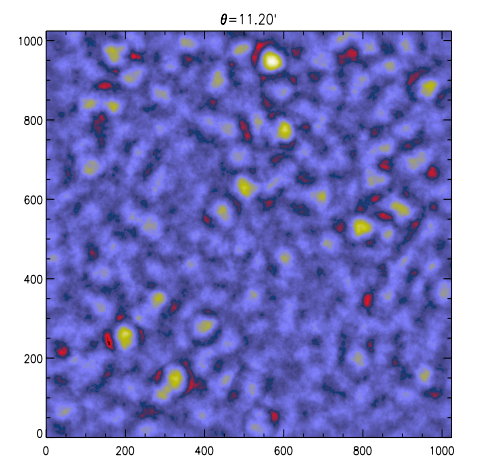Cosmological constraints from the capture of non-Gaussianity in Weak Lensing dataAuthors: S. Pires, A. Leonard,  J.-L. Starck Journal: MNRAS Year: 2012 Download: ADS | arXiv

Abstract

Weak gravitational lensing has become a common tool to constrain the cosmological model. The majority of the methods to derive constraints on cosmological parameters use second-order statistics of the cosmic shear. Despite their success, second-order statistics are not optimal and degeneracies between some parameters remain. Tighter constraints can be obtained if second-order statistics are combined with a statistic that is efficient to capture non-Gaussianity. In this paper, we search for such a statistical tool and we show that there is additional information to be extracted from statistical analysis of the convergence maps beyond what can be obtained from statistical analysis of the shear field. For this purpose, we have carried out a large number of cosmological simulations along the {\sigma}8-{\Omega}m degeneracy, and we have considered three different statistics commonly used for non-Gaussian features characterization: skewness, kurtosis and peak count. To be able to investigate non-Gaussianity directly in the shear field we have used the aperture mass definition of these three statistics for different scales. Then, the results have been compared with the results obtained with the same statistics estimated in the convergence maps at the same scales. First, we show that shear statistics give similar constraints to those given by convergence statistics, if the same scale is considered. In addition, we find that the peak count statistic is the best to capture non-Gaussianities in the weak lensing field and to break the {\sigma}8-{\Omega}m degeneracy. We show that this statistical analysis should be conducted in the convergence maps: first, because there exist fast algorithms to compute the convergence map for different scales, and secondly because it offers the opportunity to denoise the reconstructed convergence map, which improves non-Gaussian features extraction.